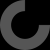MakerBot Print is our newest print-prepration software, which supports native CAD files and STL assemblies,
allows you to interact with all your printers via the Cloud, and many other exciting new features.

# Report as inappropriate

First, emmett, great work :D

I tweaked a little the OpenSCAD file to remove the line that puzzlebox_io commented.
line 260: translate ((R + R / 2-0.08) / sqrt (2) [1,1]) circle (r = R / 2);
line 261: translate ((R + R / 2-0.08) / sqrt (2)
[1, -1]) circle (r = R / 2);

Also, I changed the petal_shape module to remove a small step that appeared on the display.
line 246: color ("red") translate (1.9985 R [cos (theta) sin (theta)]) circle (r = R);
line 247: color ("orange") translate (1.9985 R [- cos (theta) sin (theta)]) circle (r = R);
line 248: color ("green") translate (1.9985 R [cos (theta) - sin (theta)]) circle (r = R);
line 249: color ("blue") translate (1.9985 R [- cos (theta) - sin (theta)]) circle (r = R);

I have not yet printed it, but at least in the visualization, errors are not displayed.

Greetings

PS: This values are for a \$fa=4.5, for others \$fa values, lines appears in visualization.